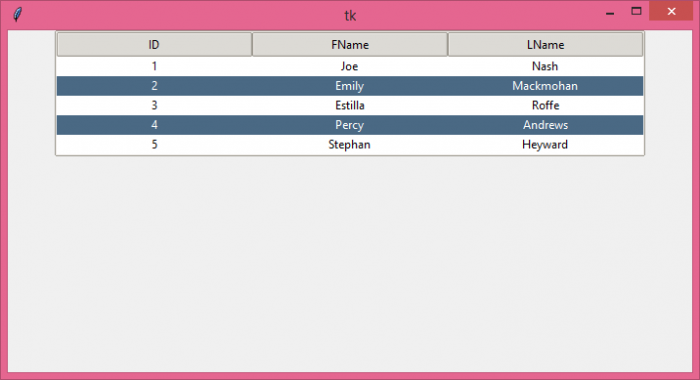# How to correctly select multiple items with the mouse in Tkinter Treeview?

The purpose of Tkinter Treeview widget is to provide the user to access the data which can be calculated and modified for the future needs of the application. The Treeview widget is used to populate the given data in a table format. We can add or insert a column, insert data into rows. Sometimes, there might be a case when we want to select multiple rows at a time. This can be done by pressing the Ctrl key and selecting the row from the table.

## Example

# Import the required libraries
from tkinter import *
from tkinter import ttk

# Create an instance of tkinter frame
win = Tk()

# Set the size of the tkinter window
win.geometry("700x350")
s = ttk.Style()
s.theme_use('clam')

tree= ttk.Treeview(win, column=("c1", "c2","c3"), show= 'headings', height= 5)
tree.column("# 1",anchor=CENTER)
tree.column("# 2", anchor= CENTER)
tree.column("# 3", anchor= CENTER)

# Insert the data in Treeview widget
tree.insert('', 'end',text= "1",values=('1', 'Joe','Nash'))
tree.insert('', 'end',text= "2",values=('2', 'Emily','Mackmohan'))
tree.insert('', 'end',text= "3",values=('3', 'Estilla','Roffe'))
tree.insert('', 'end',text= "4",values=('4', 'Percy','Andrews'))
tree.insert('', 'end',text= "5",values=('5', 'Stephan','Heyward'))

tree.pack()

win.mainloop()

## Output

Running the above code will display a table containing multiple entries in it.You can select multiple rows at a time by pressing the Ctrl key and selecting the row from the table.